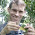## Wednesday, March 13, 2013

### Small follow-up to rate-by-state method

This is a follow-up to my earlier post on attempting to measure the influence of the state of one continuous trait on the Brownian rate of a second.

First, the posted code had a small bug. Basically there is a superfluous internal loop that does nothing (except add to the run time). This got inserted while I was programming & testing the function, and I forgot to remove it. The updated version is here.

This new version of the function also has a method, logarithm=TRUE, which fits exp(ancestors of x)~contrasts(y). This just allows for the possibility that the rate changes as a multiplicative function of x, I think.

Finally, there is a new function - sim.ratebystate - which simulates under each of the assumed models. Here's a quick demo:

> tree<-pbtree(n=200,scale=1)
> source("ratebystate.R")
> XY<-sim.ratebystate(tree,sig2x=0.1,method="by.branch", plot=TRUE,beta=c(0,2))
> ratebystate(tree,XY[,"x"],XY[,"y"],method="by.branch")
\$beta
 2.069869
\$r
 0.2875908
\$P
 0.01
\$corr
 "pearson"
\$method
 "by.branch"

I also built a new version of phytools (phytools 0.2-26) that can be downloaded and installed from source.

#### 1 comment:

1.I should say it fits contrasts(y)~exp(ancestors of x) when logarithm=TRUE.

Note: due to the very large amount of spam, all comments are now automatically submitted for moderation.